# Ncert Solutions for Class 8 Maths Chapter 12:Exponents CBSE Part 1

In this page we have NCERT book Solutions for Class 8th Maths Chapter 12:Exponents and Power for EXERCISE 1 . Hope you like them and do not forget to like , social share and comment at the end of the page.
Question 1
Evaluate
i) 3 -2
ii) (- 4) - 2
iii) (1/2)-5
As we know that
b-n= 1/bn
(i)  3-2  =  1/ 32  =1/9
(ii) (-4)-2   =1/(-4)2= 1/6
(iii) (1/2)-5   =1/ 2-5 =25= 32
Question 2
Simplify and express the result in power notation with positive exponent.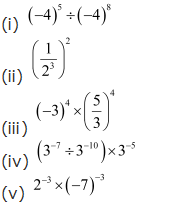(i)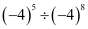= (-4)5 / (-4)8
= (-4)5-8
=1/ (-4)3 (ii)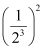=   1/23x2
=1/26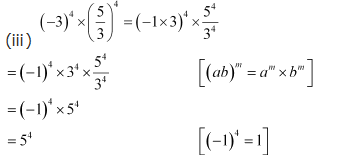iv )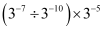=   (3-7/ 3-10) × 3-5
=3-7+10 × 3-5
=33 × 3-5
=3-2
=1/32
v) 2 - 3 × ( - 7) - 3
=   (2× -7)-3
= (-14)-3
=1/ (-14)3
Question 3
Find the value of
1. (30 + 4 - 1) × 22
2. (2 - 1 × 4 - 1)  ÷ 2 - 2
3.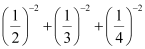4. (3 - 1 + 4 - 1 + 5 - 1)0
5.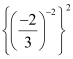1. (30 + 4 - 1) × 22 = (1+1/4) × 4
= 4+1=5
2. (2 - 1 × 4 - 1)  ÷ 2 - 2
= [(1/2) × (1/4)] ÷ (1/4)
= (1/8)  ×  (4)  =1/2
3.= 22 + 32  + 42
=4+9+16=29
4. (3 - 1 + 4 - 1 + 5 - 1)0
=1   as  a0=1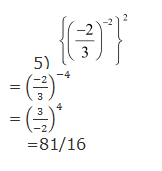Question 4
Evaluate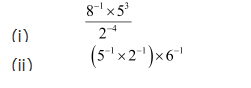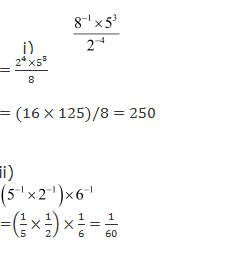Question 5
Find the value of m for which 5m   ÷  5-3 = 55

5m   ÷   5-3 = 55
5m × (1/5-3)  = 55
5m+3 =55
So  m+3=5
m=2
Question 6
Evaluate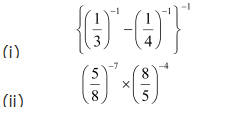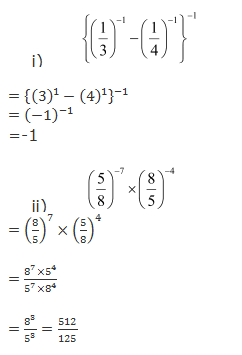Question 7
Simplify.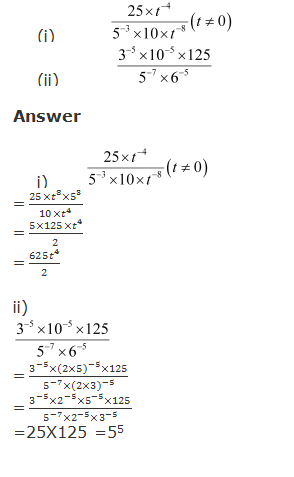Reference Books for class 8 Math

Given below are the links of some of the reference books for class 8 Math.

1. Mathematics Foundation Course for JEE/Olympiad : Class 8 This book can take students maths skills further. Only buy if child is interested in Olympiad/JEE foundation courses.
2. Mathematics for Class 8 by R S Aggarwal Detailed Mathematics book to clear basics and concepts. I would say it is a must have book for class 8 student.
3. Pearson Foundation Series (IIT -JEE / NEET) Physics, Chemistry, Maths & Biology for Class 8 (Main Books) | PCMB Combo : These set of books could help your child if he aims to get extra knowledge of science and maths. These would be helpful if child wants to prepare for competitive exams like JEE/NEET. Only buy if you can provide help to the child while studying.
4. Reasoning Olympiad Workbook - Class 8 :- Reasoning helps sharpen the mind of child. I would recommend students practicing reasoning even though they are not appearing for Olympiad.

You can use above books for extra knowledge and practicing different questions.

Note to our visitors :-

Thanks for visiting our website.